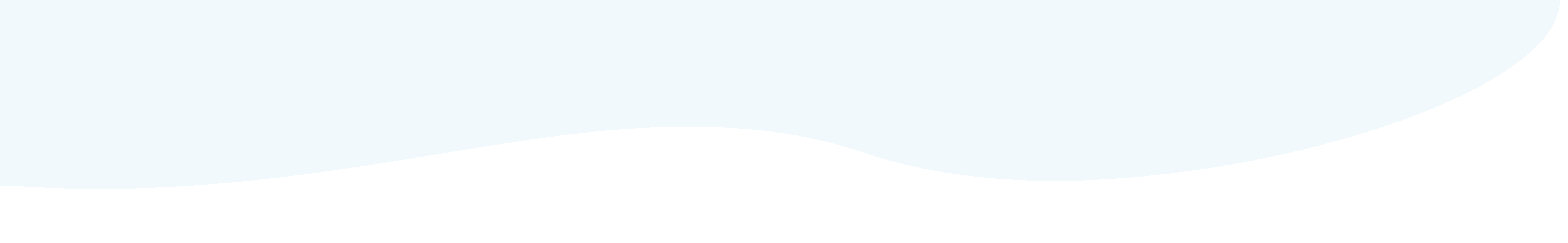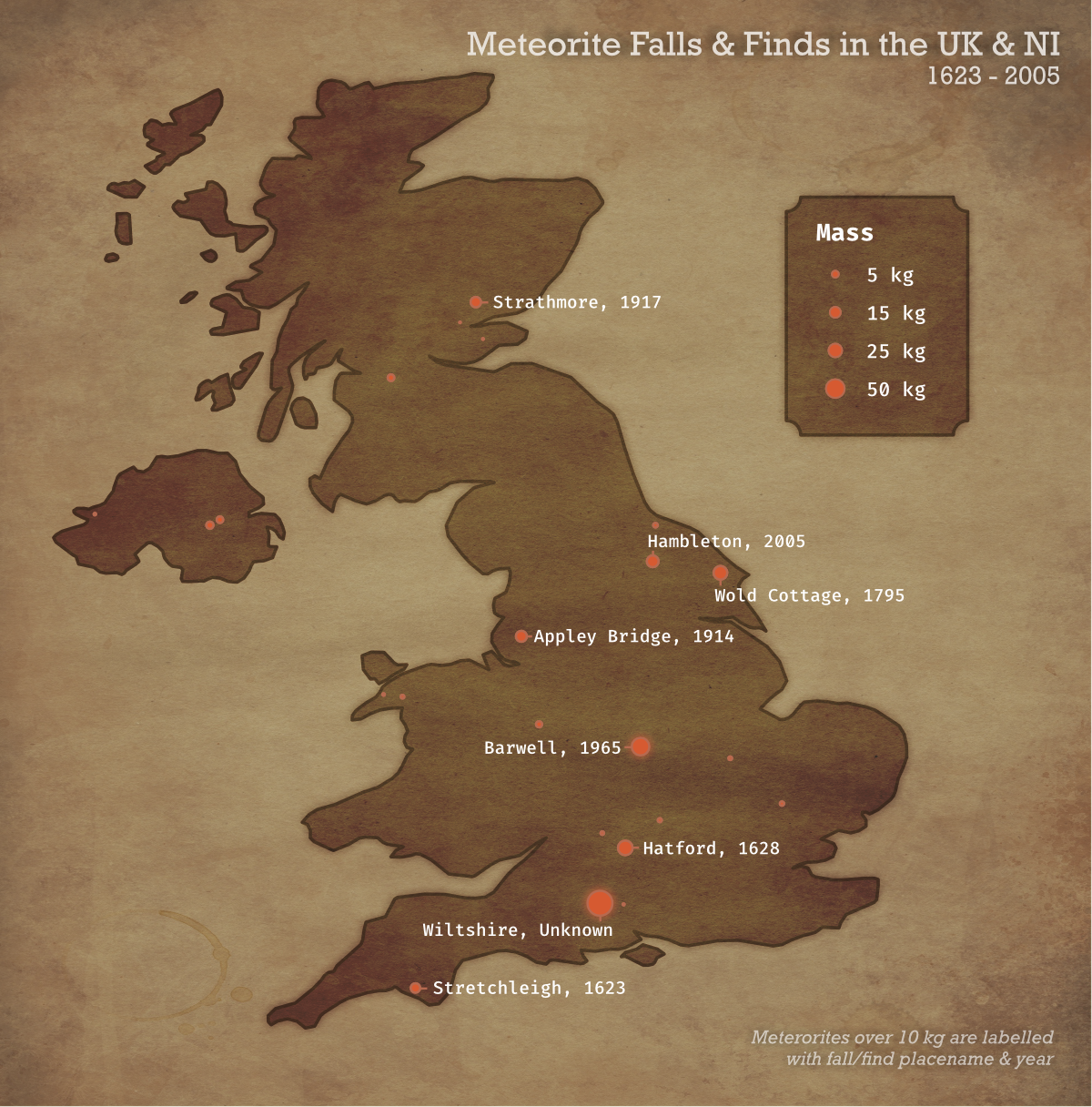# Meteorite Falls and Finds in the UK

(Tidy Tuesday is a project to supply weekly data sets for R users to practice their coding skills on. You can find full details here.)

The Tidy Tuesday dataset for this week, compiling meteorite strikes across the world, grabbed my interest so I decided to finally dive in and give it a go. I ended up looking at strikes in the UK, noted by mass.I exported the original code image as a PDF and then applied some effects in Affinity Photo to give it an old, weathered look. This was my first time experimenting with something like this, so it’s a little sketchy, but I enjoyed seeing how I could pull a plot apart in Photo to apply image effects (although sorry, this does mean the code for this plot doesn’t get you all the way to the finished produced).

Code:

``````library(tidyverse)
library(maps)

ukFalls <- meteorites %>%
filter(lat >= 50 & lat <= 60 & long <= 2 & long >= -11) %>%
filter(!name %in% c("Limerick", "Dundrum", "Mooresfort", "Leighlinbridge", "Pettiswood")) %>%
mutate(massKG = mass/1000) %>%
mutate(nameYear = paste(name, year, sep = ", ")) %>%
arrange(year)

ukMap <- map_data(regions = "uk", map = "world") %>% filter(lat <=58.73)

ggplot(ukMap, aes(x = long, y = lat)) +
geom_polygon(aes(group = group), colour = "black", fill = "#222831") +
geom_point(data = ukFalls, aes(x = long, y = lat, size = massKG), colour = "#d65a31") +
geom_text(data = subset(ukFalls, massKG >=10), aes(label = nameYear), hjust = 0, nudge_x = 0.2, size = 3) +
scale_size(range = c(0, 5), breaks = c(5,15,25,50), labels = c("5 kg", "15 kg", "25 kg", "50 kg")) +
theme_void() +
theme(plot.background = element_rect(fill = "#5588a3"),
plot.margin = margin(.5, .5, .5, .5, "cm")) +
labs(size = "Mass",
alpha = "Mass")

ggsave("map.pdf")``````